# PI Function in Excel

Returns the mathematical constant called PI

## What is the PI Function in Excel?

The PI Function in Excel returns the mathematical constant “pi.” To recap, PI is the ratio of a circle’s circumference and its diameter. Often, we would use the PI Function in Excel, especially when our business is required to do geometric calculations. These calculations entail calculating the area of a new office space, a new factory, etc.

When a human does a PI calculation, it will approximate PI as 3.14. However, Excel stores the value of PI accurately to 15 digits and up to 14 decimal places.

### Formula for the PI Function

The syntax for the PI function is = PI()

In Excel, if you just input = PI(), you will get the value of PI as shown below: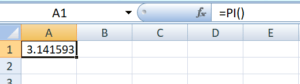### How to do calculations using the PI Function in Excel?

Let’s take an example to understand it. Let’s assume we are given 8 as the radius and are required to calculate the area of the circle. The formula to use would be:1. To do, this you would first open your Excel spreadsheet. Now input A1 = 8 (radius).
2. The next step would be to input the formula in the adjacent cell as shown below: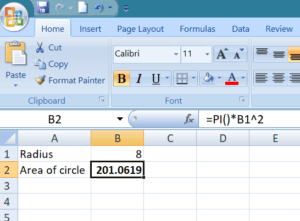Remember the PI function takes no arguments. The PI function, as we all are aware in Excel, stores the value up to 15 decimals. However, as seen above, the answer is only up to four decimals. What happened here was that Excel rounded up the answer to correspond with the cell’s degree of accuracy. We can increase the number of decimal places as required. As shown below, I raised it to 15 decimal places. This was done by going to Home tab > Format button > Format Cells. The Format Cells dialog would by default display the Number tab.

Now select Number under the category and then type 14 into the Decimal Places field to use all 14 digits of the constant PI in the formula. Click OK.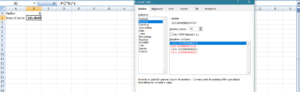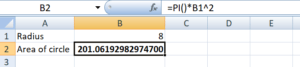### PI Examples in VBA

You can also use the PI function in VBA. Type:

application.worksheetfunction.PI()

For the function’s arguments, you can either enter them directly into the function or define the variables to use instead.

### Free Excel Course

If you want to learn more about functions and become an expert on them, check out our CFI’s Free Excel Crash Course!  Go through our step by step instructions and demonstrations on how to become an Excel power user.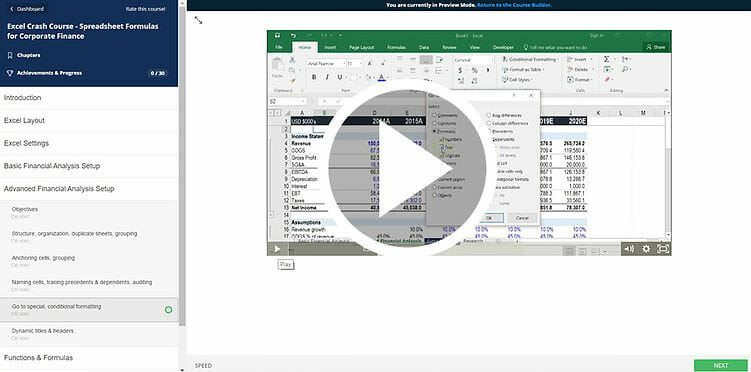Thanks for reading CFI’s guide to important Excel functions! By taking the time to learn and master these functions, you’ll significantly speed up your financial analysis. To learn more, check out these additional CFI resources:

• Excel Functions for Finance